# What is a LOT in Forex Trading? - Lot Sizes Explained

Updated May 08 2022

What is a LOT in Forex Trading? - Lot Sizes Explained

A LOT is a measure to efficiently communicate standardized quantities of currency transactions, it's far easier to say “1 LOT” than saying “One hundred thousand U.S Dollars”.

A Standard LOT in Forex Trading equals to 100.000 units of any given currency. For example, 1 Standard LOT of EUR/USD equals to €100.000.

Other lot sizes commonly used are:

• Mini LOT (also referred as 0.1 lot) - 10.000 units of any given currency.
• Micro LOT (also referred as 0.01 lot) - 1.000 units of any given currency.
• Nano LOT (also referred as 0.001 lot) - 100 units of any given currency.

The objective of using this terminology is to facilitate communication and reduce errors when conducting Forex transactions. Here's what you'll learn in this guide:

## Featured Low Spreads Forex Brokers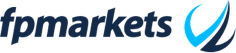75.31% of retail CFD accounts lose money.

Dynamic - From 0 to 1.2 Pips71.24% of retail CFD accounts lose money.

Dynamic - From 0 Pips to 1.3 Pips74-89% of retail CFD accounts lose money.

From 0.4 Pips to 1.61 Pips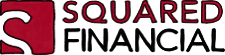63.2% of retail CFD accounts lose money.

Dynamic - From 0 to 1.2 Pips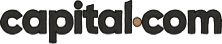83.45% of retail CFD accounts lose money. Trading on margin is high risk and is not suitable for everyone.

Dynamic - From 0.8 Pips

## PIP Value per Lot SizeA PIP is the smallest price measurement change in a currency trading. In the case of EUR/USD a PIP is worth 0.0001, in the case of USD/JPY a PIP is worth 0.01.

• If EUR/USD is trading at 1.1456 and prices change to 1.1457 we can say that it moved 1 PIP.
• If USD/JPY is trading at 114.22 and prices change to 114.23 we can say that it moved 1 PIP.

The objective of using PIP values per Lot is to determine:

• How much money are we profiting or losing per each PIP that our trade moves taking into account the quantity of currency that we chose to trade with.

In the form of a question it’s the same as if you asked yourself: if I go long on EUR/USD with 5 Lots (€500.000), how much money am i profiting or losing every time EUR/USD changes 0.0001 in price?

The PIP value per LOT size answers this question and does so with a result expressed using the base currency, then you can convert it into whatever currency you desire.

### PIP Value per Lot Size Formula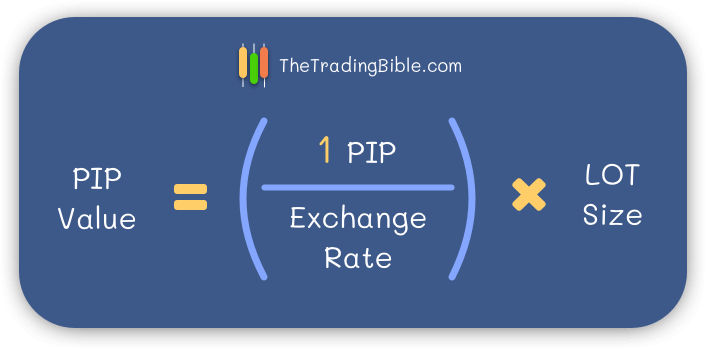• The first part of the formula is doing a simple currency conversion, we are dividing our PIP value according to the pair we trade (0.0001 or 0.01) by the current exchange rate, that way we know how much is that PIP worth in terms of the currency we are trading.
• The second part is multiplying that result by the LOT size we are trading (100.000, 10.000, 1.000, 100) to understand the impact of that previous number we determined in the total amount of currency units we are trading with.

### PIP Value per Lot Size Example

Let’s create an example together, we’ll use 1 Mini LOT of USD/JPY.

• 1 Mini Lot ( also referred to as 0.1 Lot) equals 10.000 units of currency.
• Our Base currency in USD/JPY is the USD, so this transaction is for \$10.000 worth of Japanese Yens.
• The current value for USD/JPY is 103.84

Formula execution goes as follows: ( 0.01/103.84)*10.000 and the result is \$0.96, that is the PIP value for our Mini Lot (0.1 Lot) sized trade.

This means that for each PIP that the USD/JPY pair moves (0.01 in price change) then we’re either profiting or losing \$0.96.

Important:

In the example above, the Base currency was USD, so the result of our formula is of course in USD.

If you were to run the formula on a pair where the Base currency was EUR then the result of the formula is expressed in EUR, so if you want to handle all your calculations in USD then converting the result is as simple as multiplying the result of your formula by the EUR/USD exchange rate at the moment, and then you’ll realise another interesting fact:

When the Quote currency is the USD, these calculations are usually not necessary as you’ll always arrive at the same results for the PIP per Lot size value when you perform the conversion of your formula’s result into U.S Dollars.

Here are two Examples:

PIP value in a standard lot of EUR/USD - Current Trading price 1.2227 - Let’s apply our formula:

• (0.0001/1.2227)*100.000 = 8.1786 (this result is in EUR, our base currency)
• Converting the result of our formula to US Dollars by multiplying our result by the current EUR/USD exchange rate
• 8.1786 * 1.2227 = \$10

PIP value in a standard lot of GBP/USD - Current Trading price 1.3469 - Let’s apply our formula:

• (0.0001/1.3469)*100.000 = 7.4244 (this result is in GBP, our base currency)
• Converting the result of our formula to US Dollars by multiplying our result by the current GBP/USD exchange rate
• Converting the result to US Dollars: 7.4244  * 1.3469 = \$10

Now you know, we always arrive at the same final result when the quote currency is the US Dollar.

## Standard Lot in Forex

A standard lot size equals 100.000 units of any given currency. Usually this trade size is already considered big and requires a lot of care when calculating the pip value. Let’s look at some examples:

• 1 LOT OF EUR/USD equals to a €100.000 purchase worth of U.S Dollars
• 1 LOT OF USD/JPY equals to a \$100.000 purchase worth of Japanese Yens

### PIP Value per Standard Lot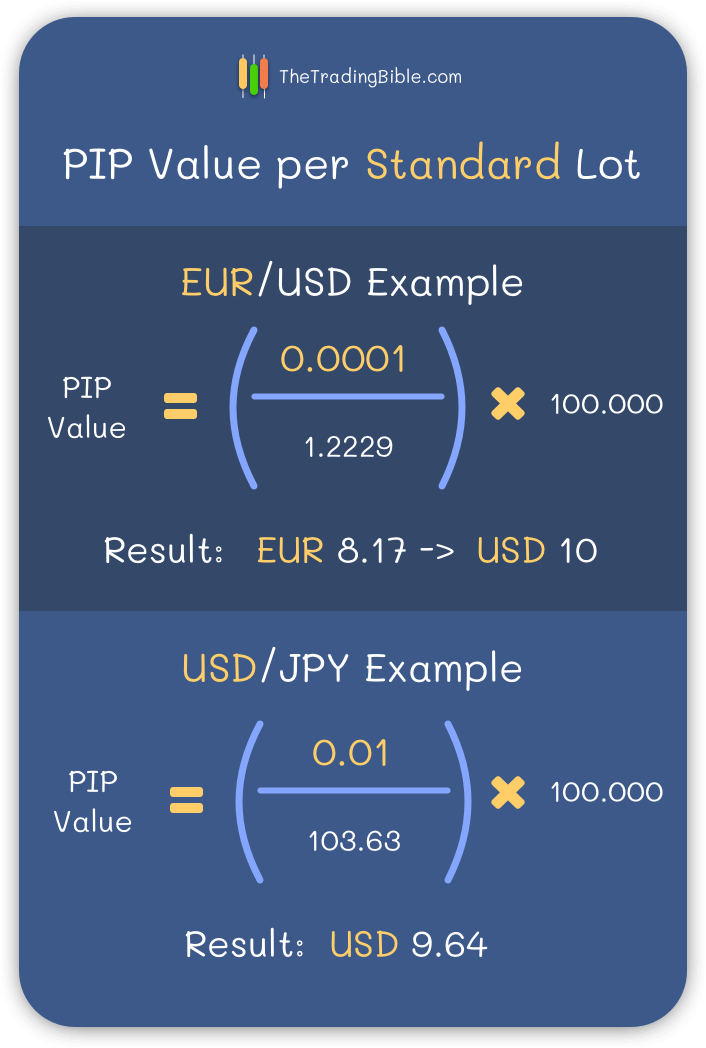In any currency pair where the USD is the quote (the second currency) the PIP value per Standard lot is \$10

For any other case, apply the formula we explained earlier and you’ll get the result expressed in the currency of the base unit.

If your base currency was the US Dollar, then you already got your result expressed in US Dollars. If your base currency was any other, you can convert the result of your formula to any other currency you choose.

## Mini Lot in Forex

A Mini LOT size equals 10.000 units of any given currency. A Mini lot can also be referred to as 0.1 Lot. Here are some examples:

• 1 Mini LOT of EUR/USD equals to a €10.000 purchase worth of U.S Dollars
• 0.1 LOT of USD/JPY equals to a \$10.000 purchase worth of Japanese Yens

### PIP Value per Mini Lot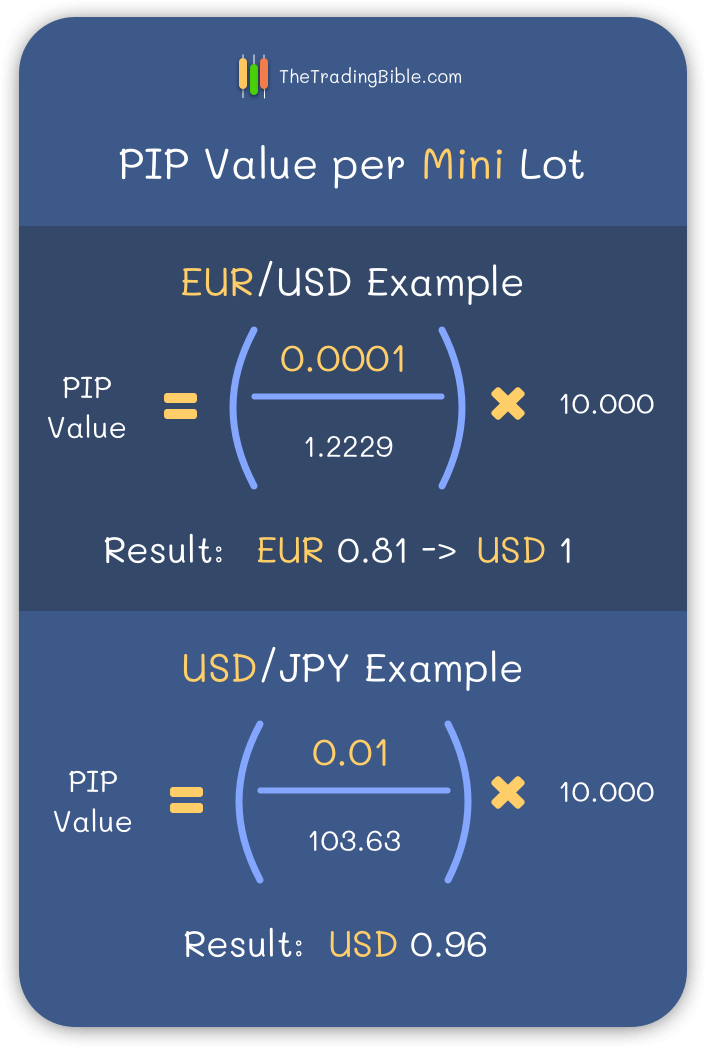In any currency pair where the USD is the quote (the second currency) the PIP value per Mini lot is \$1

For any other case, apply the formula we explained earlier and you’ll get the result expressed in the currency of the base unit.

If your base currency was the US Dollar, then you already got your result expressed in US Dollars. If your base currency was any other, you can convert the result of your formula to any other currency you choose.

## Micro Lot in Forex

A Micro LOT size equals 1000 units of any given currency. A Micro lot can also be referred to as 0.01 Lot. Here are some examples:

• 1 Micro LOT of EUR/USD equals to a €1000 purchase worth of U.S Dollars
• 0.01 LOT of USD/JPY equals to a \$1000 purchase worth of Japanese Yens

### PIP Value per Micro Lot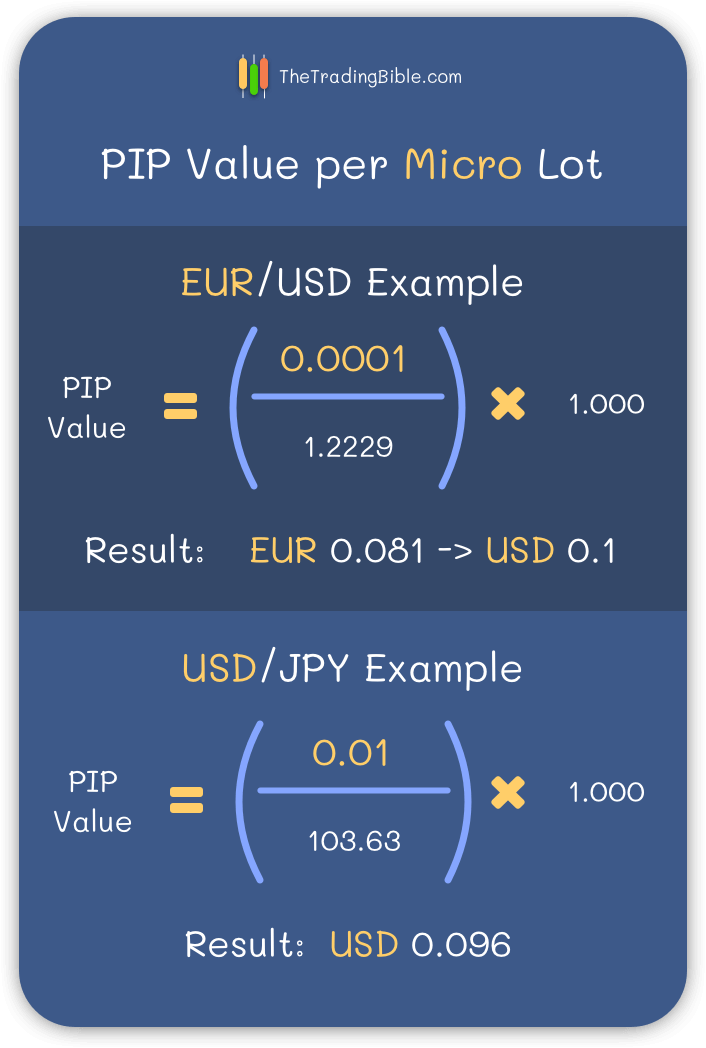In any currency pair where the USD is the quote (the second currency) the PIP value per Micro lot is \$0.1

For any other case, apply the formula we explained earlier and you’ll get the result expressed in the currency of the base unit.

If your base currency was the US Dollar, then you already got your result expressed in US Dollars. If your base currency was any other, you can convert the result of your formula to any other currency you choose.

## Nano Lot in Forex

A Nano LOT size equals 100 units of any given currency. A Nano lot can also be referred to as 0.001 Lot. Here are some examples:

• 1 Nano LOT of EUR/USD equals to a €100 purchase worth of U.S Dollars
• 0.001 LOT of USD/JPY equals to a \$100 purchase worth of Japanese Yens

### PIP Value per Nano Lot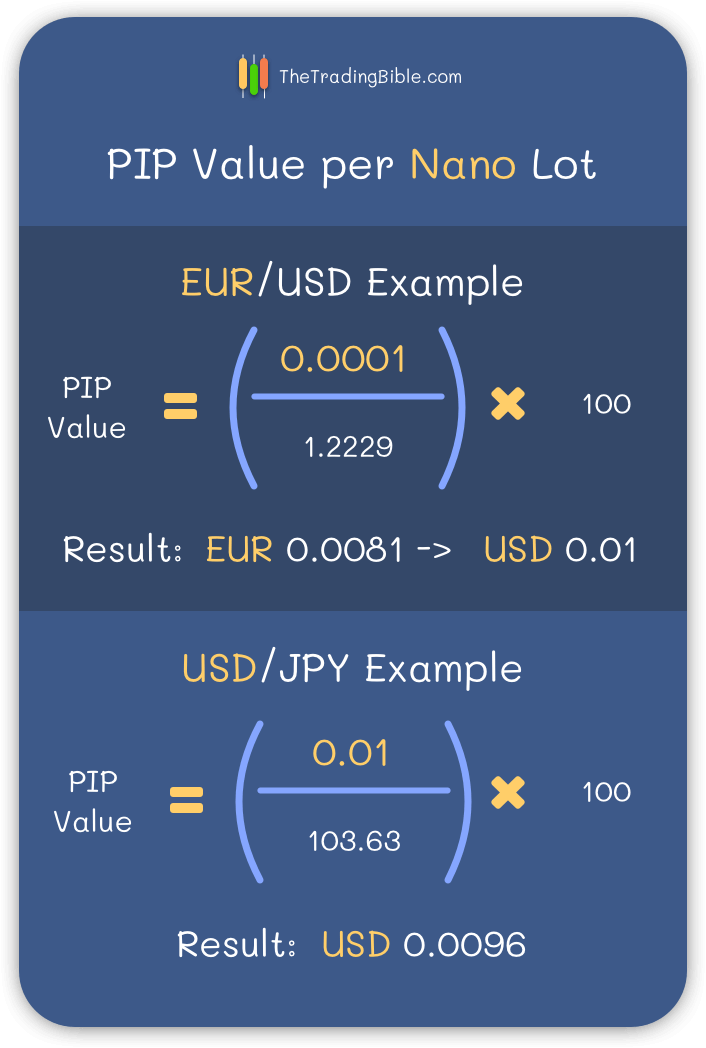For any other case, apply the formula we explained earlier and you’ll get the result expressed in the currency of the base unit.

If your base currency was the US Dollar, then you already got your result expressed in US Dollars. If your base currency was any other, you can convert the result of your formula to any other currency you choose.

## Conclusion

Knowing the different lot sizes available and how to calculate the pip per lot size value, will allow you to develop efficient risk management plans when trading.

If you know that any given currency fluctuates 100 PIPS per day and your risk management plan fits a max daily loss of \$100 then you wouldn’t open a Standard lot trade, right? That would expose you to a huge profit/loss potential outside your risk management plan.

This is the mistake that many new traders make due to brokers providing high leverage factors to all their clients, when a a newbie trader sees that he can open a position worth \$100.000 (1 standard lot) using \$100 as margin requirement, just because he cans he does so and this couldn’t be more wrong, remember to always fit every single trade in your risk management plan.

When researching to write this article we noticed that other websites focus on providing tables that you can memorise for certain pip values per lot sizes and we didn’t like that. It will make you dependent on always looking at a table and not knowing how to arrive at such mathematical results by yourself without needing the help of anyone.

Good luck!

### How much is 0.1 Lot Size in Forex?

0.1 Lots in Forex equals to 10.000 currency units, which is also called a Mini Lot. To achieve this result all you need to do is multiply 0.1 by 100.000 (the standard lot value).

### How much is 0.01 Lot Size in Forex?

0.01 Lots in Forex equals to 1.000 currency units, which is also called a Micro Lot. To achieve this result all you need to do is multiply 0.01 by 100.000 (the standard lot value).

### How much is 0.001 Lot Size in Forex?

0.001 Lots in Forex equals to 100 currency units, which is also called a Nano Lot. To achieve this result all you need to do is multiply 0.001 by 100.000 (the standard lot value).

### How much is 100 Lots in Forex?

100 Lots in Forex amounts to 10.000.000 currency units. To achieve this result you need to multiply 100 by 100.000 (the standard lot value).

### USD/JPY Lot Size

Since Lots are always expressed on the base currency (the first one) and we know that a standard lot is 100.000 currency units, that means that a standard lot of USD/JPY is equivalent to \$100.000 worth of Japanese Yens.

Author

Stefano TrevisoFeatured Brokers

175.31% of retail CFD accounts lose money.

271.24% of retail CFD accounts lose money.

374-89% of retail CFD accounts lose money.

463.2% of retail CFD accounts lose money.

5